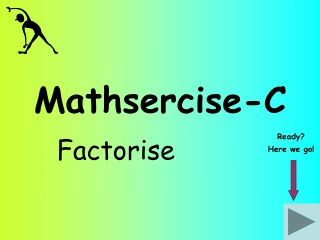DownloadDownload PresentationMathsercise-C

# Mathsercise-C

Télécharger la présentation## Mathsercise-C

- - - - - - - - - - - - - - - - - - - - - - - - - - - E N D - - - - - - - - - - - - - - - - - - - - - - - - - - -
##### Presentation Transcript

1. Mathsercise-C Factorise Ready? Here we go!

2. Factorise….. Find the common factor(s) in all terms. In this case it is 4! 12x + 4 Question 2 12x + 4 4 4 (3x + 1) Notice that this is 1 and not 0! Answer 1 Factorising

3. Find the common factor(s) in all terms. In this case it is 6! Factorise …. 6x + 18y Question 3 6 (x + 3y) 6 6x + 18y Answer 2 Factorising

4. Find the common factor(s) in all terms. In this case it is 4x! Factorise…… 8xy + 12x Question 4 4x 8xy + 12x 4x (2y + 3) Answer Why is this just 3? 3 Factorising

5. Factorise….. Find the common factor(s) in all terms. In this case it is 3x! 6x2 – 3xy Start 6x2 – 3xy (2x – y) 3x 3x Answer 4 Factorising